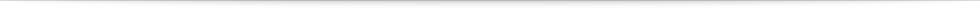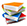Teach
With
Bigshot
 Prev Introduction      Gears      Gearbox      Electromagnetism Dynamo      Battery      Circuit      Illustrations Next### Gears

 Figure 2: Two intermeshed gears

Gears are used in almost every machine with spinning parts − in wristwatches, bicycles, cars and even trains. A gear is generally cylindrical in shape with grooves − called teeth or cogs − cut along its boundary . Two gears with identical teeth can mesh together perfectly. Figure 2 shows two intermeshed gears. One gear's teeth lock between the other’s so that turning the first gear causes the second one to turn as well (click on the play button).

Any two gears working together this way provide “mechanical advantages” that have made them useful in all kinds of machines. Depending on their relative sizes, one gear can either increase or decrease the speed of rotation of the other. In addition, they can be used to reverse the direction of rotation. Observe that in Figure 2 the two gears A and B rotate in opposite directions. Moreover, gear B spins faster than gear A. The ratio of their speeds is called the gear ratio.

How fast does B spin compared to A? Since the teeth on both gears must be of the same size for the gears to intermesh, the smaller gear has fewer teeth than the larger one. Notice that by the time the smaller gear B makes a complete rotation (all of its teeth have passed by the point P), the larger gear A would not have completed a full rotation (not all of its teeth will have passed by P). In other words, the smaller gear spins faster than the bigger one. It can be shown  that the speeds of the two gears, A and B, are related by the following equation:

Speed of A × Number of teeth on A = Speed of B × Number of teeth on B.

The number of teeth on a gear depends on its outer boundary, or circumference. The gear's circumference, in turn, is proportional to its diameter. Therefore, we can write the above equation as:

Speed of A × Diameter of A = Speed of B × Diameter of B.

For example, in Figure 2, the diameter of gear A is 3 times that of gear B, which means that gear B rotates 3 times faster.

 Prev Next###References

  N. Karim, "How Gears Work" HowStuffWorks.com. Nov 16, 2000. Available: http://science.howstuffworks.com/transport/engines-equipment/gear.htm  D. W. Dudley, Handbook of Practical Gear Design. CRC, 1994.